# Light

Light is the form of energy that can be found in nature from the sources like sun and other artificial sources.

Lens: lens is transparent refracting medium bonded by two spherical surfaces. There are two types of lens:

Concave lens: This lens is thin at its centre than at edges. Diverging lens is also called concave lens. It is thinner in the middle and thicker at the edges and has virtual focus.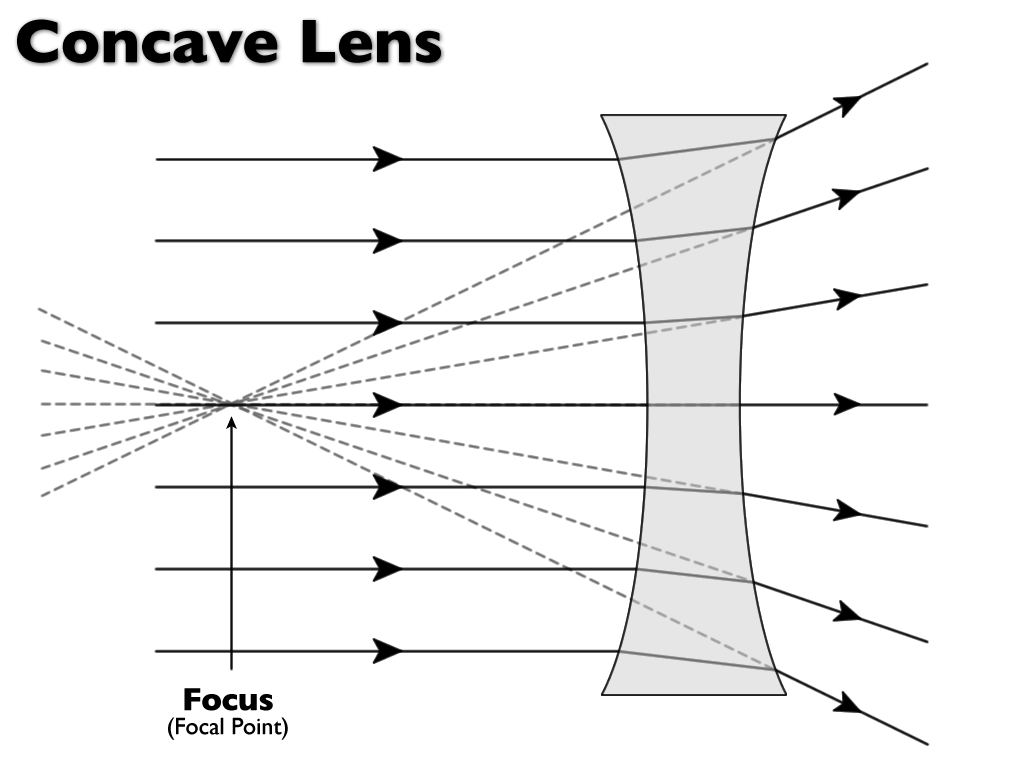Convex lens: This lens is thick at its centre and thin at its edges .A convex lens is called converging lens because it converge rays of light passing through it at a point after refraction.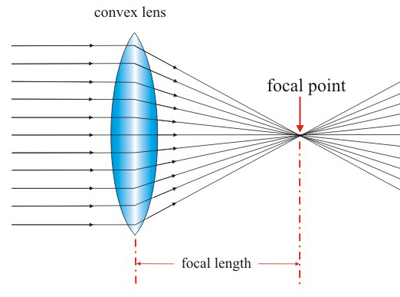Principal axis:

It is the straight line passing through the two centre of curvature.

Optical centre:

The geometrical centre of lens is called optical centre.

Focal length:

It is the distance between the optical centre and principal focus.

Principal focus:

When rays parallel to the principal axis of a concave (or convex) lens appear to diverge (or converge) from a point then it is called principal focus.

Real image and virtual image:

Image that are formed on the screen are the real image.eg image formed by the convex lens.

Image that are not formed on the screen are the virtual image formed by concave lens.

Ray diagram of the convex lens:

a. Parallel rays coming from the infinity meet at the focus after refraction by convex lens. The nature of image is real inverted and diminished.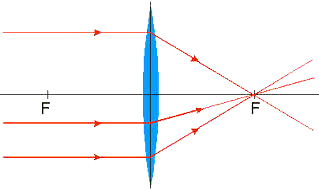b.When the object is placed beyond the 2F, real inverted and diminished image is formed between F and 2F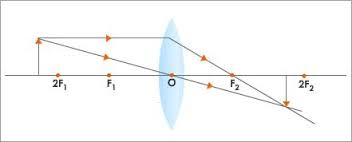c. When the object is placed at 2F, the nature of the image   is real, inverted and of the same size.d. When the object is placed between 2F and F the image is formed beyond the 2F which is real, inverted and magnified.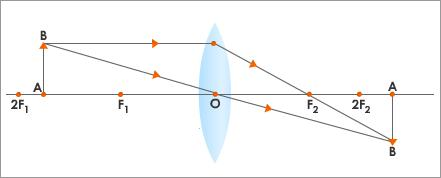e. When the object is placed at focus the image is formed at infinity which is real inverted and magnified.f.When the object is kept at optical centre and focus, virtual magnified and erect is formed.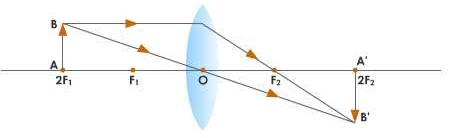Ray diagram of the concave lens:

When the object is kept between infinity and focus, virtual erect and diminished image is formed between the focus and optical centre on the same side of the object in the lens.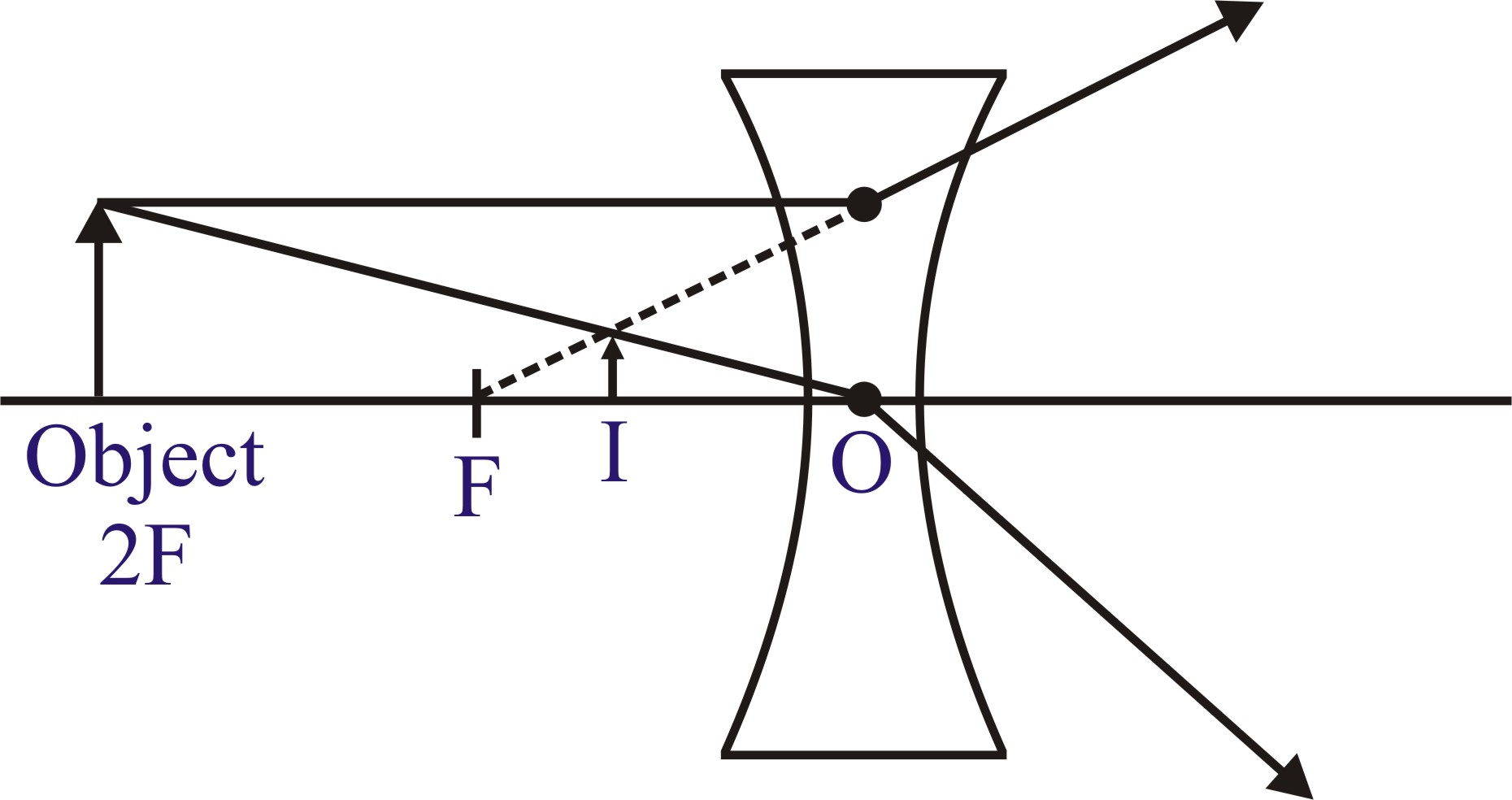When the object is kept infinity, virtual erect and highly diminished image is formed at focus.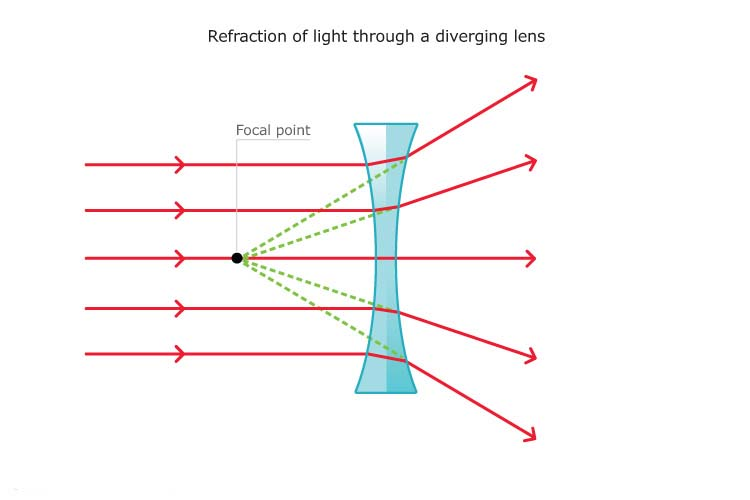Magnification:

The ratio of the size of the image to the size of the object is called magnification.

M = I/O =(height of image) height of object= Image distance (v)/object distance (u)

Relation between focal length, object distance and image distance:

The formula for lens is:
1/focal length(f) = 1/object distance(u)+1/image distance(v)

Power of lens:

The power of lens is defined as the ability of a lens to converge or diverge light rays falling on it.

Power(P) = 1/f

The S.I unit of the power is dioptre.

Optical instruments:

Those instruments which use lenses, prism, and mirror are called optical instruments.

Power of accommodation:

The ability of eye to change the focal length so that image is always formed at retina is called power of accommodation.

The nearest point which eye can see is called near point and it is 25cm.

The farthest point which eye can see is called far point and it is infinity.

Defect of vision:

The inability of the eye to form a image on retina of the object lying between the far and near point is called defect of vision.

Long sightedness (hypermetroia):

The defects of eye in which a person can see object at farther distance but cannot see the object at close distance is known as long sightedness.Causes:

Shortening of eye ball

Inability of ciliary muscles of the eyes press for   the required thickness, when the eye lens becomes thin

Remedy:

By using the convex lens of suitable focal length.

Short sightedness (myopia):

Short sightedness is the defect in which the person has the ability to see the object at near distance but has weakness in seeing the object at farther distance.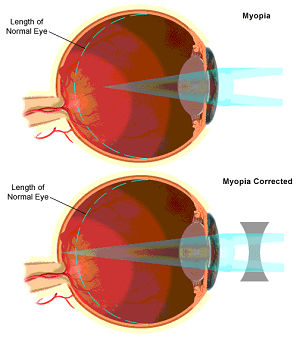Causes:

Inability of ciliary muscles of the eye to press for the required thickness when the eye lens becomes thick

Elongation of eyeball

Remedy:

By using the concave lens of suitable focal length.

Microscope:

Microscope is the optical instrument which is used to view image of the small object in a magnified form.There are two types of microscope

Simple microscope

Compound microscope

Telescope:

Telescope is the optical instrument, which is used to view the distantobject. There are two types telescope:

Astronomical telescope

Terrestrial telescope